# RD Sharma Solutions for Class 12 Maths Chapter 12 Higher Order Derivatives

## RD Sharma Solutions for Class 12 Maths Chapter 12 – Free PDF Download Updated for 2023-24

RD Sharma Solutions for Class 12 Maths Chapter 12 – Higher Order Derivatives are primarily designed for CBSE Class 12 students and are based on the latest syllabus prescribed, as per the CCE guidelines by the CBSE Board. Students are required to thoroughly go through RD Sharma Solutions while solving problems from the RD Sharma textbook before the board exams to score well and intensify their problem-solving abilities. These solutions are prepared by experts in Maths at BYJU’S to provide exercise-wise solutions in a precise manner.

RD Sharma Solutions also include tips and tricks to solve difficult problems quickly and with much confidence. By practising RD Sharma Solutions for Class 12 regularly, students will be thorough with the concepts provided in the Class 12 RD Sharma textbook. Students can access RD Sharma Solutions for Class 12 Maths Chapter 12 Higher Order Derivatives from the below provided links. This chapter mainly focuses on relations involving various order relations of cartesian functions.

## RD Sharma Solutions Class 12 Maths Chapter 12 Higher Order Derivatives:

### Access answers to Maths RD Sharma Solutions For Class 12 Chapter 12 – Higher Order Derivatives

Exercise 12.1 Page No: 12.17

1. Find the second order derivatives of the each of the following functions:

(i) x3 + tan x

Solution: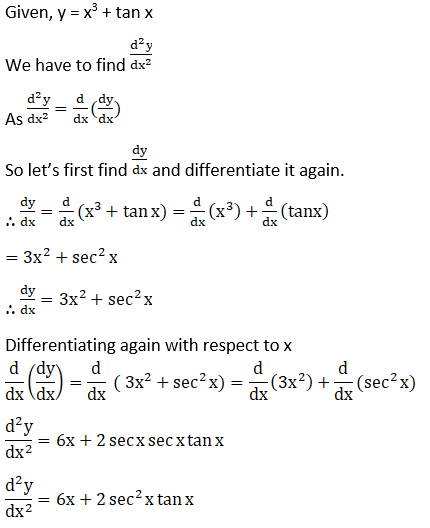(ii) Sin (log x)

Solution: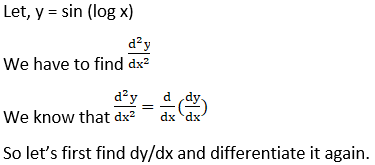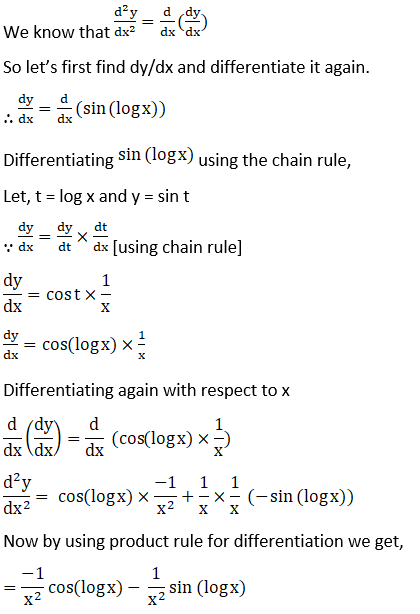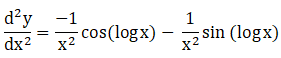(iii) Log (sin x)

Solution: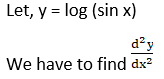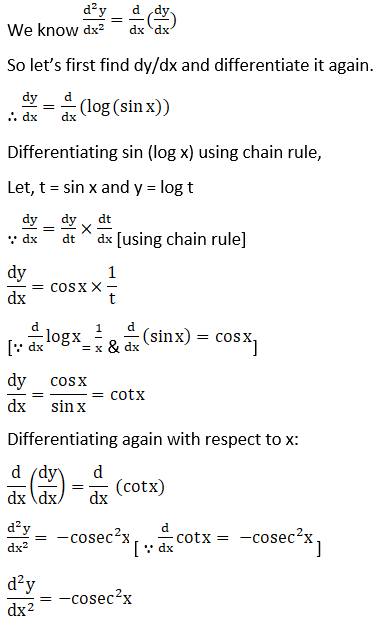(iv) ex sin 5x

Solution: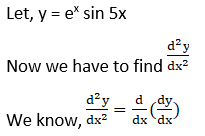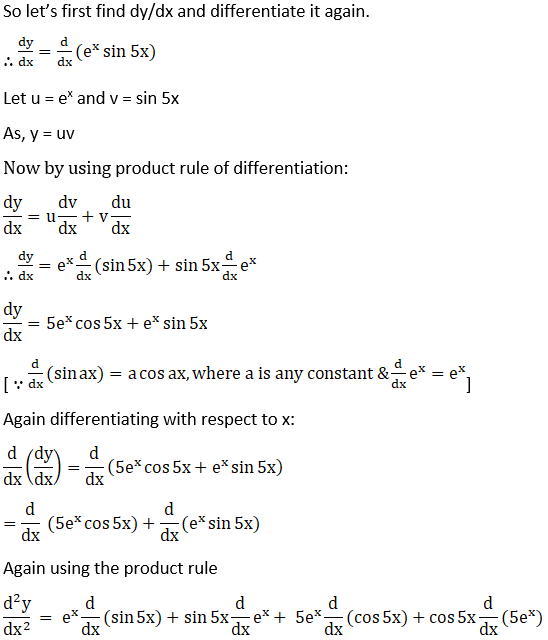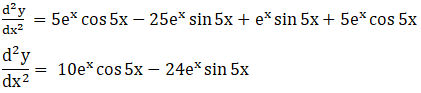(v) e6x cos 3x

Solution:

Let, y = e6x cos 3x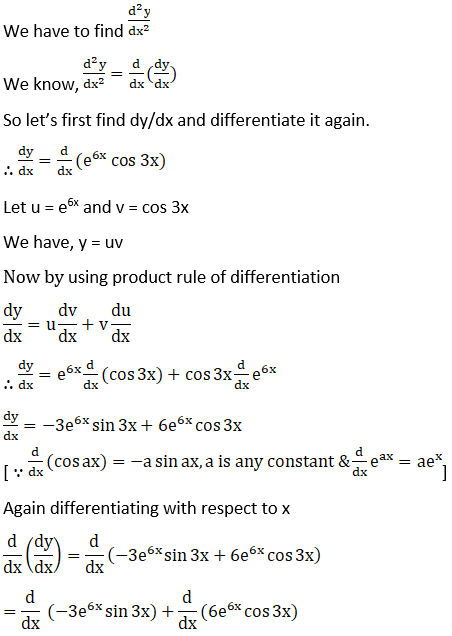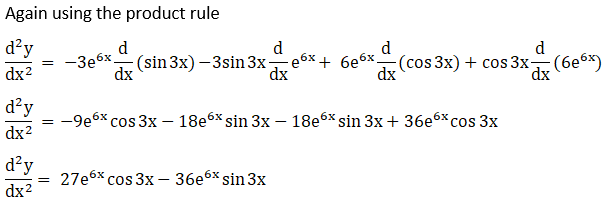(vi) x3 log x

Solution: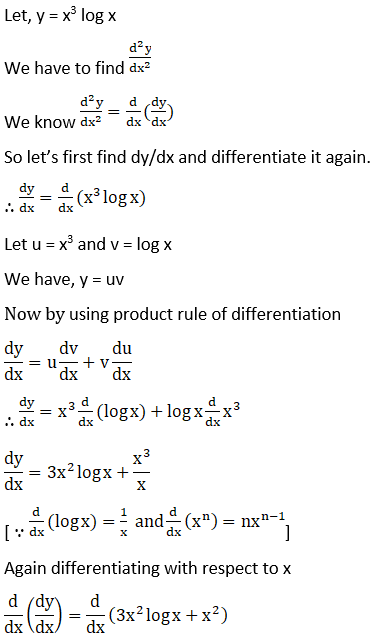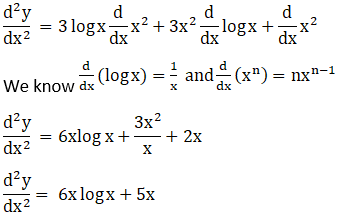(vii) tan-1x

Solution: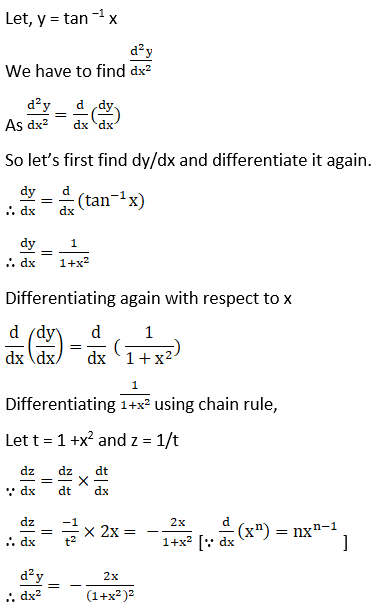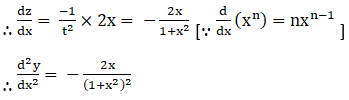(viii) x cos x

Solution: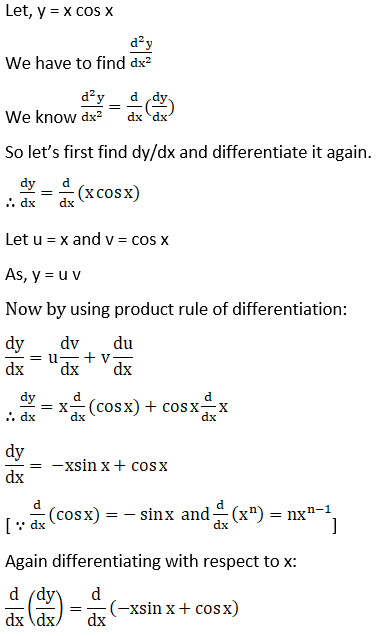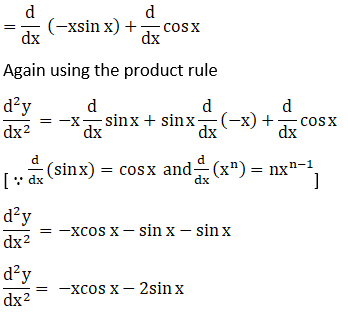(ix) Log (log x)

Solution: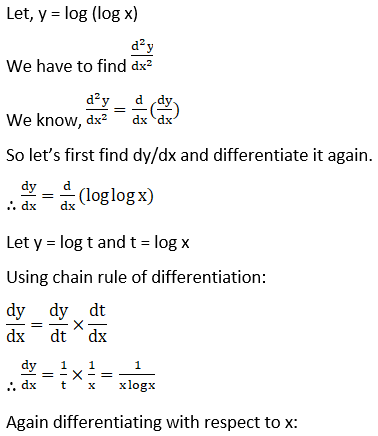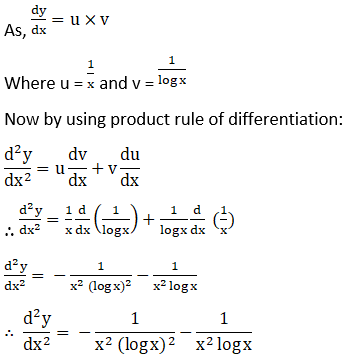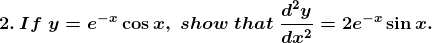Solution: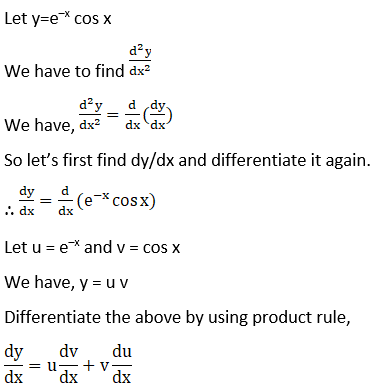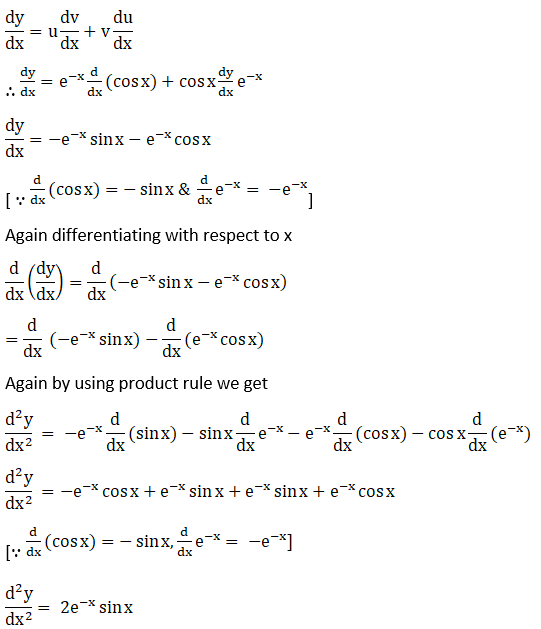Hence proved.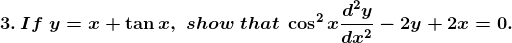Solution:

Given y = x + tan x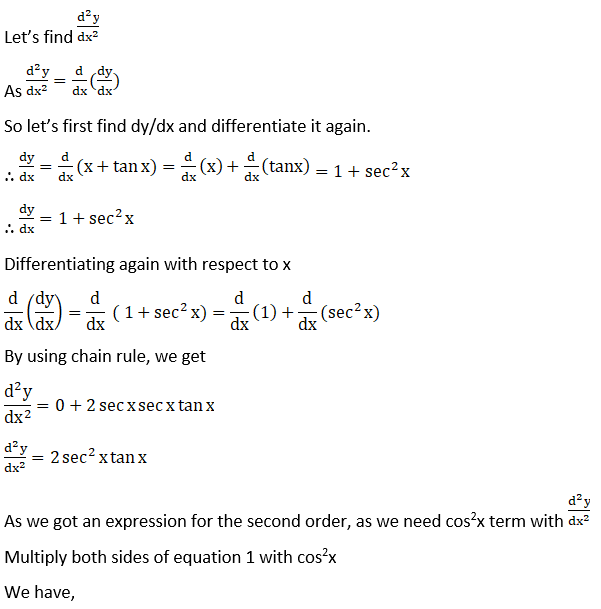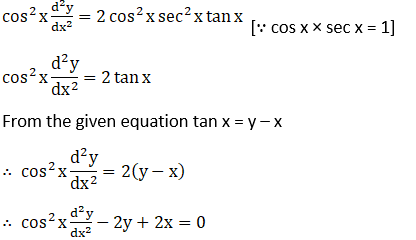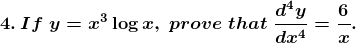Solution: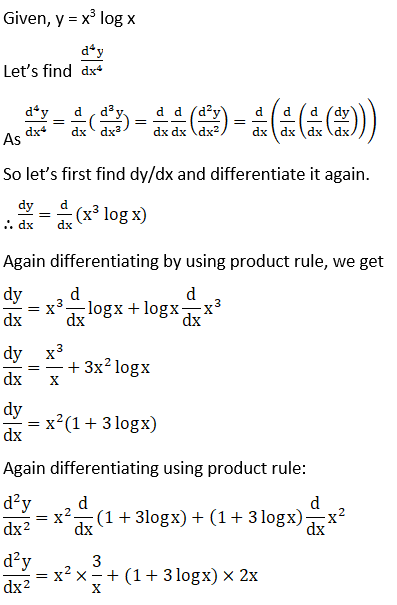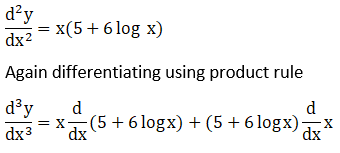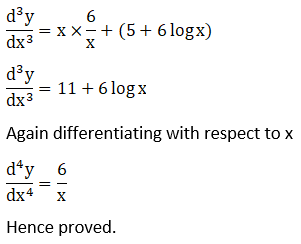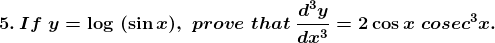Solution: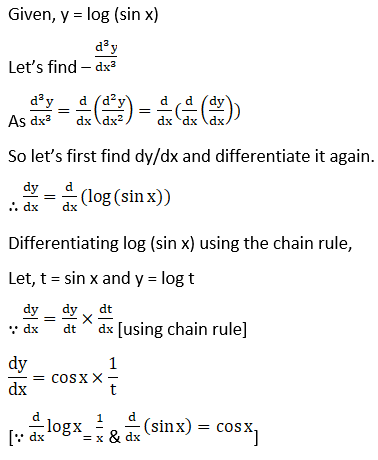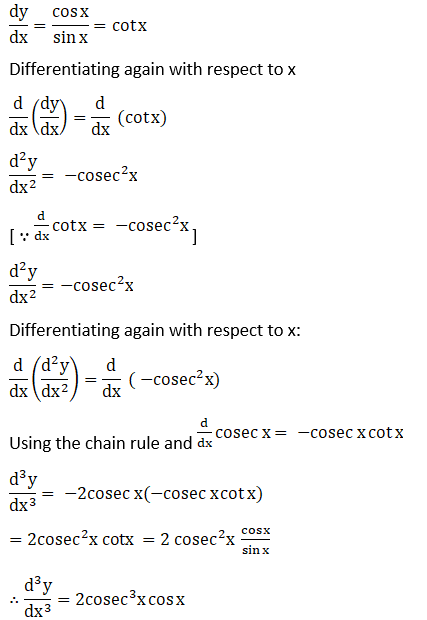Hence proved.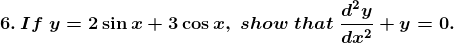Solution: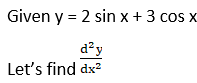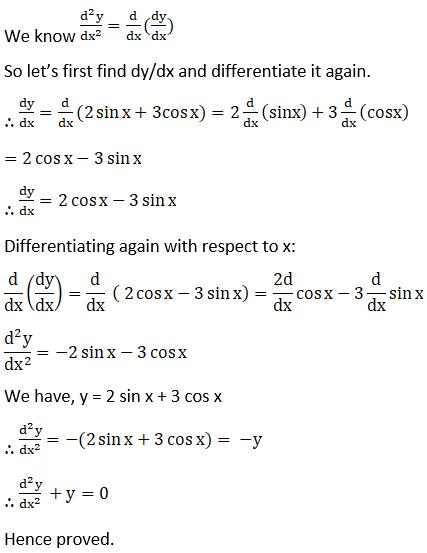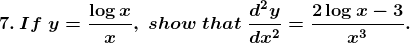Solution: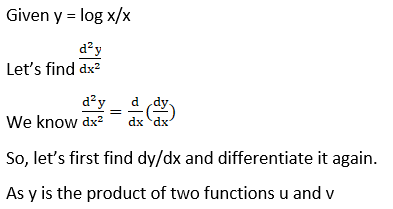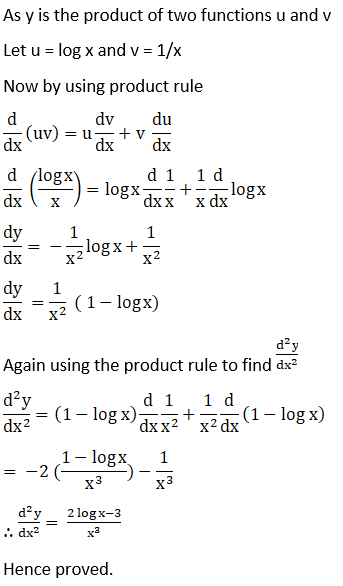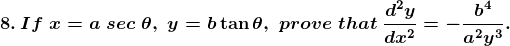Solution: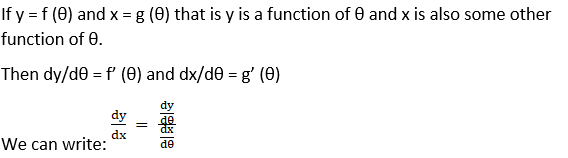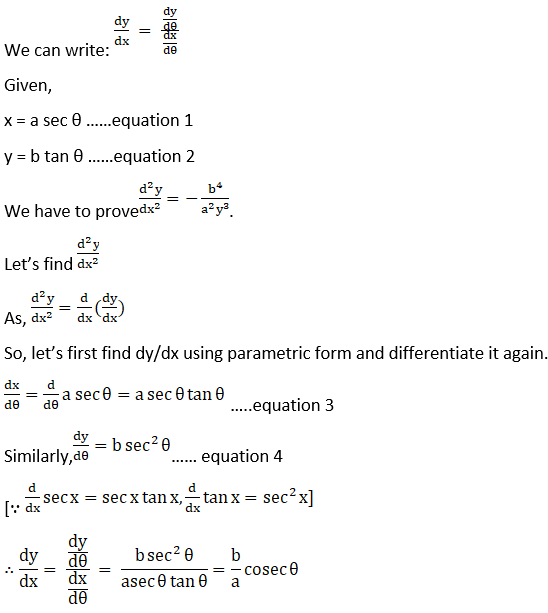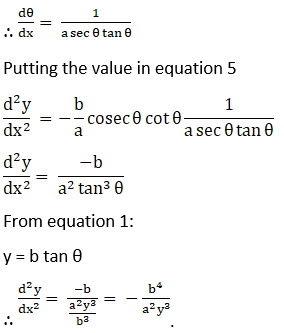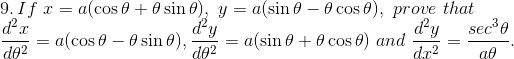Solution: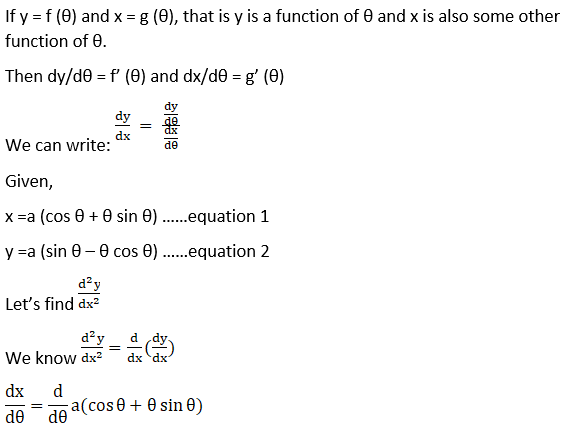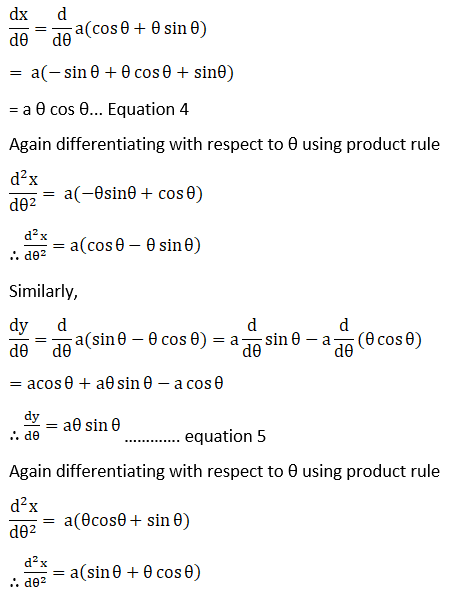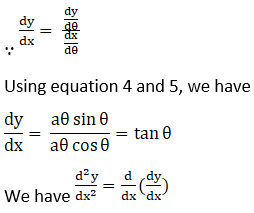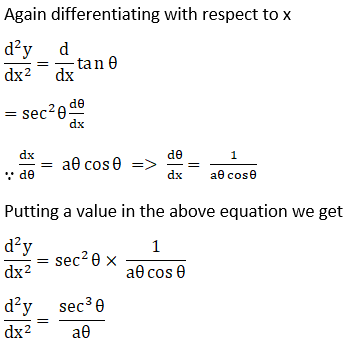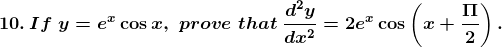Solution: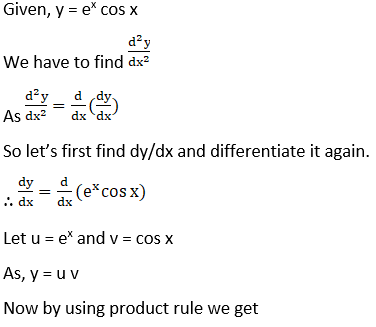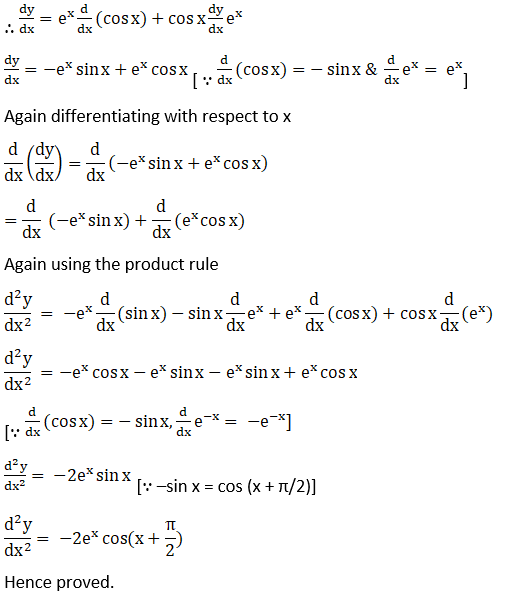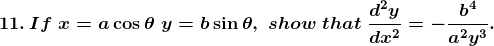Solution: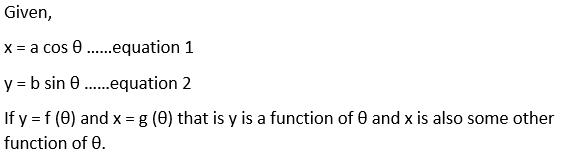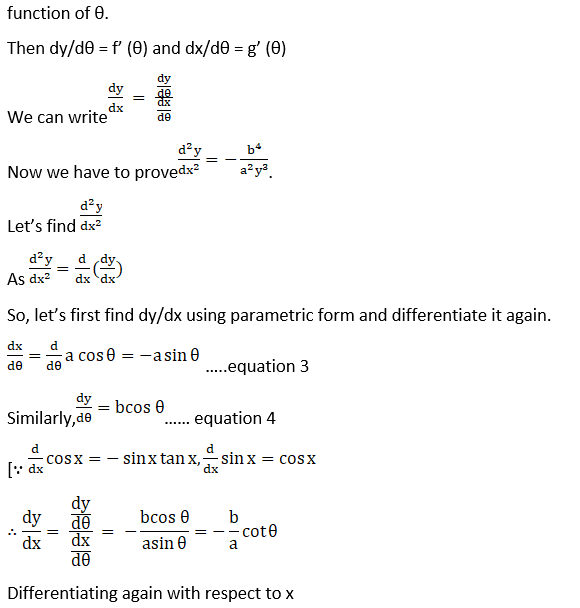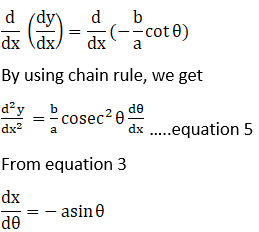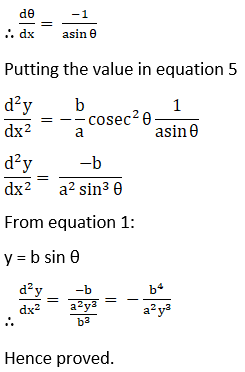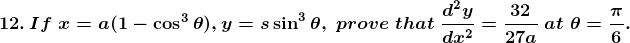Solution: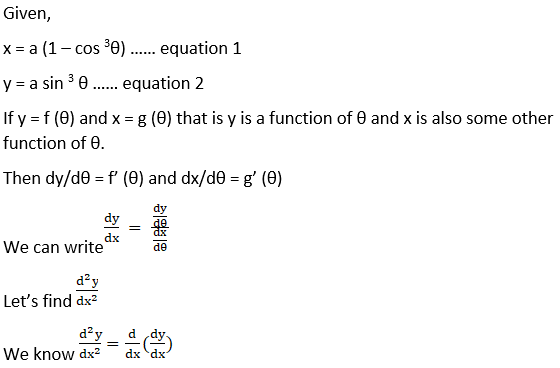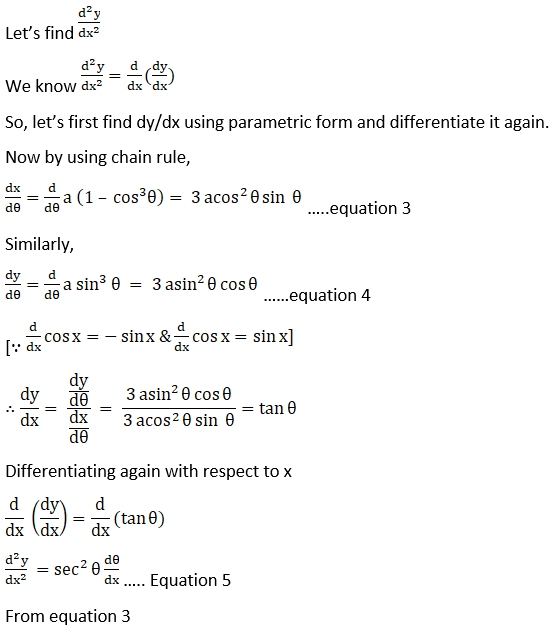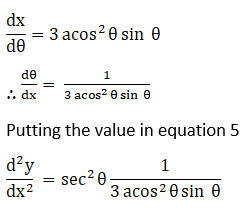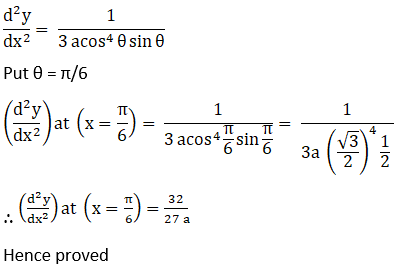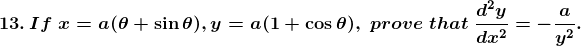Solution: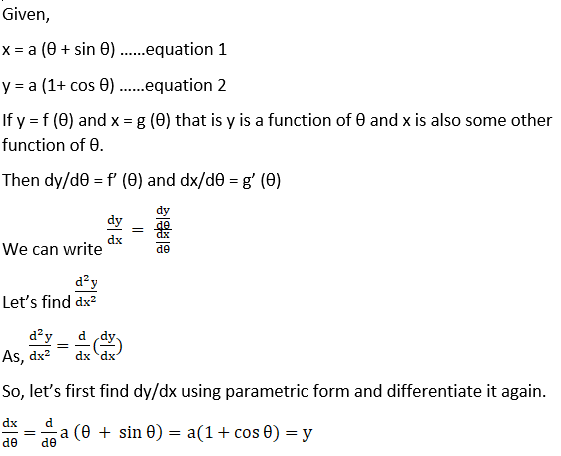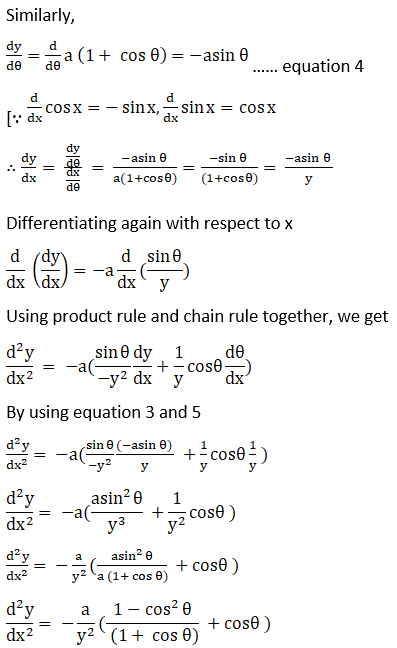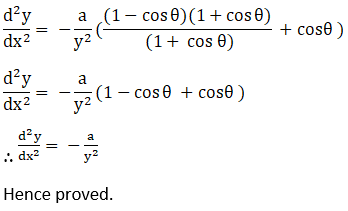Hence proved.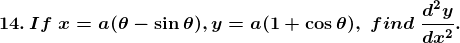Solution: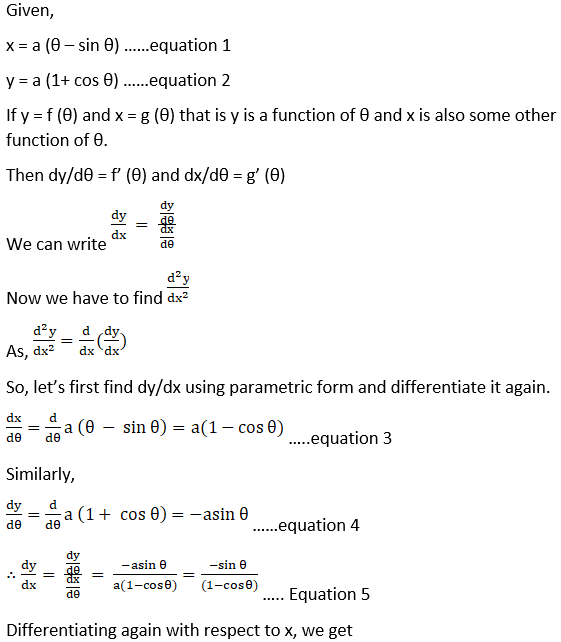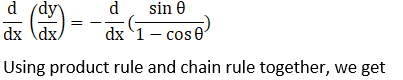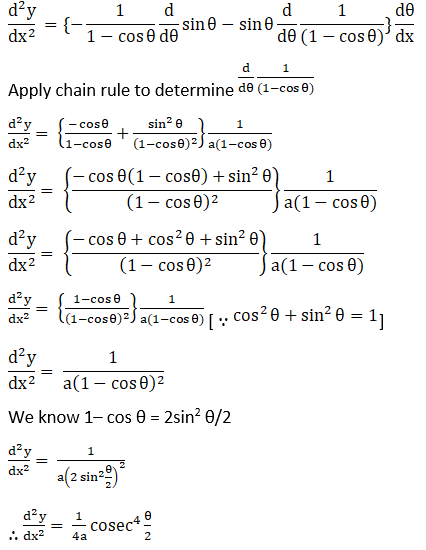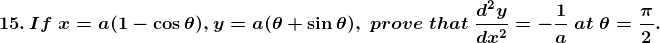Solution: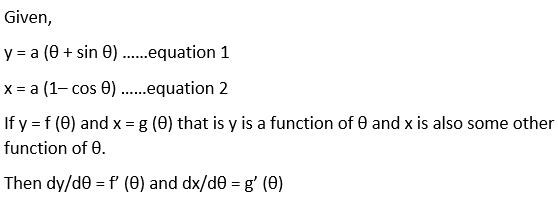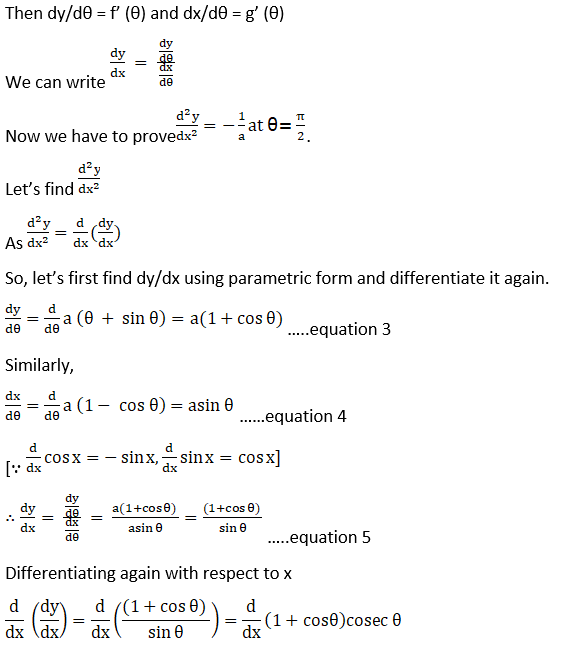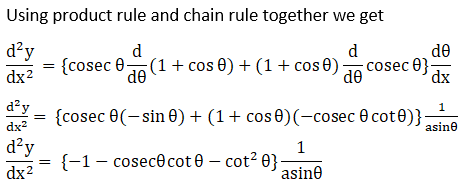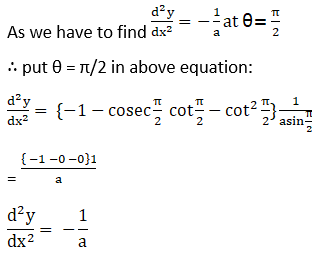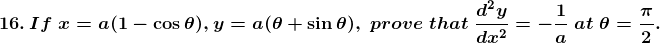Solution: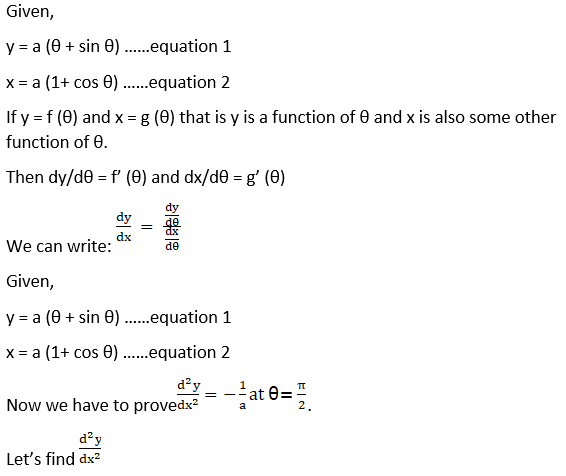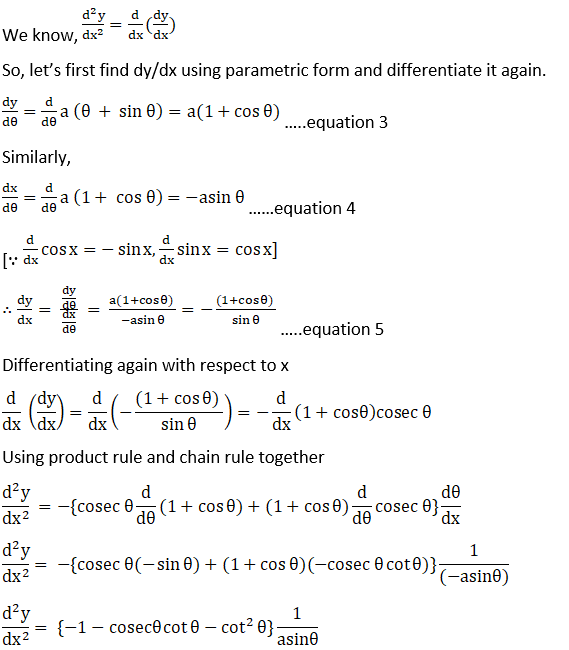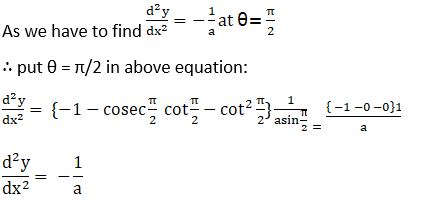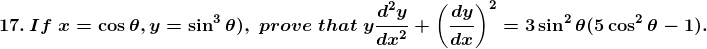Solution: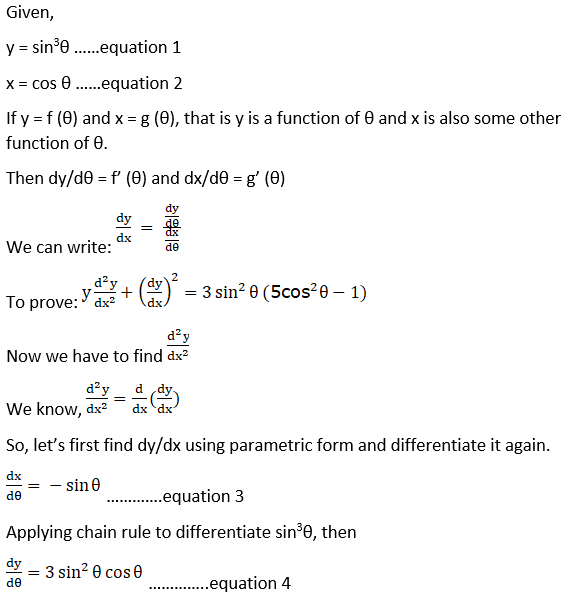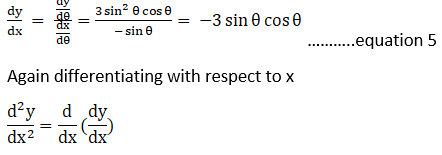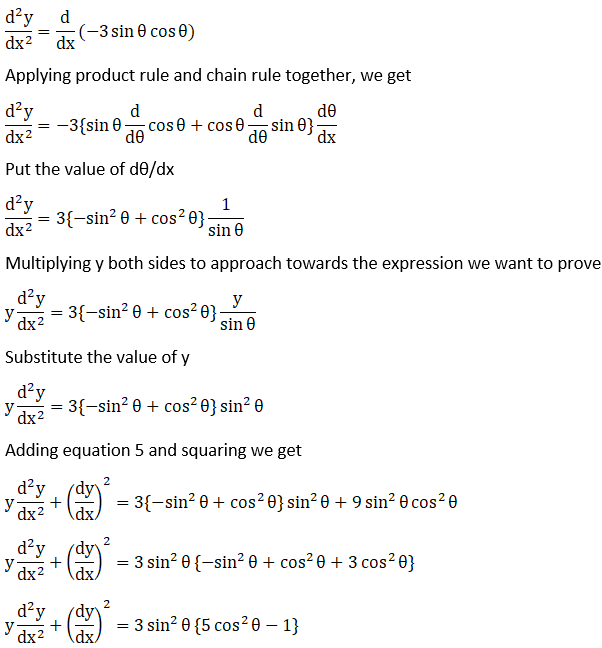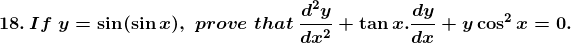Solution: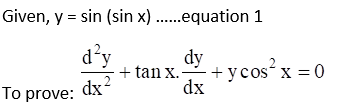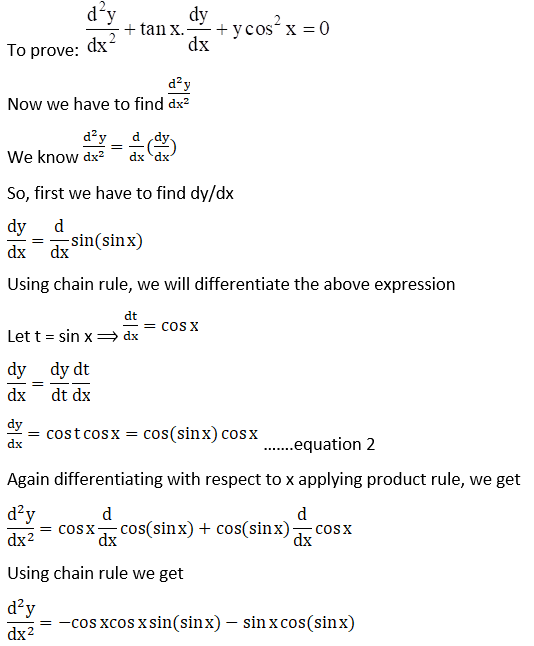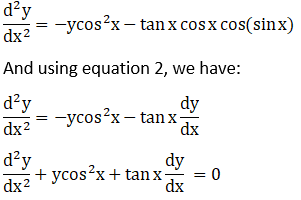## RD Sharma Class 12 Maths Solutions Chapter 12 Higher Order Derivatives

Some of the essential topics of this chapter are listed below:

• Definition of higher-order derivatives.
• Notations of higher-order derivatives.
• Proving relations involving various order derivatives of cartesian functions.
• Finding second-order derivatives of parametric functions.
• Proving relations involving various order derivatives of parametric functions.
• Proving relations involving various order derivatives.

## Frequently Asked Questions on RD Sharma Solutions for Class 12 Maths Chapter 12

Q1

### Is the RD Sharma Solutions for Class 12 Maths Chapter 12 sufficient for the CBSE students?

Students under the CBSE board are advised to choose the best study material available in the current market. The RD Sharma Solutions from BYJU’S are created by subject experts with the main aim of helping students to score well in the board exams. The answers are prepared in an understandable language to make learning fun for the students. The solutions help in clarifying the queries of students which arise while learning the chapter.
Q2

### Is BYJU’S providing solutions for RD Sharma Class 12 Maths Chapter 12?

Yes, BYJU’S website provides accurate and detailed solutions for all questions provided in the textbook. The RD Sharma Solutions for Class 12 Maths Chapter 12 can be downloaded in the form of a PDF and students can use it as a reference tool to quickly review all the questions. BYJU’S brings you RD Sharma Solutions for Class 12 Maths Chapter 12, designed by our subject experts to facilitate a smooth and precise understanding of chapters.
Q3

### Can the RD Sharma Solutions for Class 12 Maths Chapter 12 be considered as the best study material for exam preparations?

We, at BYJU’S, have provided solutions to all the textbook questions based on the latest CBSE guidelines. All the solutions are framed by individual subject-matter experts to help students ace the exam. The answers are compiled in PDFs so that students can download them for free and refer to them anywhere and anytime. The solutions contain detailed explanations in a step-by-step manner to boost the confidence of students in answering the difficult type of questions efficiently.
Q4

### How do the RD Sharma Solutions for Class 12 Maths Chapter 12 enhance performance of students in board exams?

RD Sharma Solutions for Class 12 Maths Chapter 12 implements answers in an efficient manner as per the current syllabus prescribed by the CBSE board. Diligent practice of these solutions helps students build a good preparation for all sorts of questions that would be asked in the board exams. These solutions are the best material for students who aspire to obtain proficiency in solving complex problems with ease.

Q5

### Do the RD Sharma Solutions for Class 12 Maths Chapter 12 help you clear board exams with good marks?

Yes, the RD Sharma Solutions for Class 12 Maths Chapter 12 is one of the significant chapters to score optimum marks in the board exams. The solutions are designed by experts with the aim to provide students with the best study material for quick and easy calculations. Regular practice of these solutions enables students to obtain an interest in learning the covered concepts thoroughly. Solving the textbook problems using RD Sharma Solutions undoubtedly helps students ace the board exams.